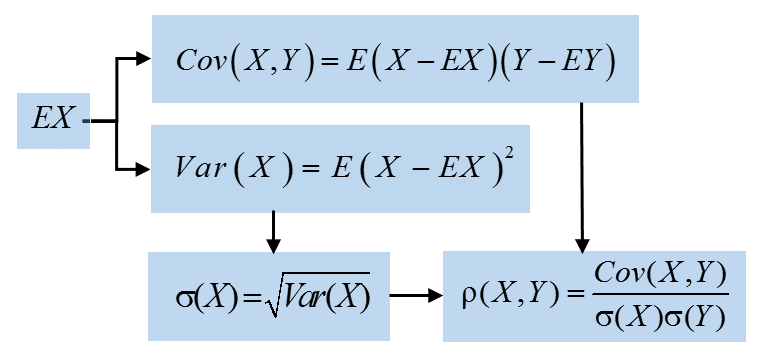26
Nov 16

## Properties of correlation

### Correlation coefficient: the last block of statistical foundation

Correlation has already been mentioned in

Statistical measures and their geometric roots

Properties of standard deviation

The pearls of AP Statistics 35

Properties of covariance

The pearls of AP Statistics 33

### The hierarchy of definitions

Suppose random variables$X,Y$ are not constant. Then their standard deviations are not zero and we can define their correlation as in Chart 1.Chart 1. Correlation definition

### Properties of correlation

Property 1. Range of the correlation coefficient: for any$X,Y$ one has$- 1 \le \rho (X,Y) \le 1$.
This follows from the Cauchy-Schwarz inequality, as explained here.

Recall from this post that correlation is cosine of the angle between$X-EX$ and$Y-EY$.
Property 2. Interpretation of extreme cases. (Part 1) If$\rho (X,Y) = 1$, then$Y = aX + b$ with$a > 0.$

(Part 2) If$\rho (X,Y) = - 1$, then$Y = aX + b$ with$a < 0$.

Proof. (Part 1)$\rho (X,Y) = 1$ implies
(1)$Cov (X,Y) = \sigma (X)\sigma (Y)$
which, in turn, implies that$Y$ is a linear function of$X$:$Y = aX + b$ (this is the second part of the Cauchy-Schwarz inequality). Further, we can establish the sign of the number$a$. By the properties of variance and covariance$Cov(X,Y)=Cov(X,aX+b)=aCov(X,X)+Cov(X,b)=aVar(X)$,$\sigma (Y)=\sigma(aX + b)=\sigma (aX)=|a|\sigma (X)$.
Plugging this in Eq. (1) we get$aVar(X) = |a|\sigma^2(X)$ and see that$a$ is positive.

The proof of Part 2 is left as an exercise.

Property 3. Suppose we want to measure correlation between weight$W$ and height$H$ of people. The measurements are either in kilos and centimeters${W_k},{H_c}$ or in pounds and feet${W_p},{H_f}$. The correlation coefficient is unit-free in the sense that it does not depend on the units used:$\rho (W_k,H_c)=\rho (W_p,H_f)$. Mathematically speaking, correlation is homogeneous of degree$0$ in both arguments.
Proof. One measurement is proportional to another,$W_k=aW_p,\ H_c=bH_f$ with some positive constants$a,b$. By homogeneity$\rho (W_k,H_c)=\frac{Cov(W_k,H_c)}{\sigma(W_k)\sigma(H_c)}=\frac{Cov(aW_p,bH_f)}{\sigma(aW_p)\sigma(bH_f)}=\frac{abCov(W_p,H_f)}{ab\sigma(W_p)\sigma (H_f)}=\rho (W_p,H_f).$